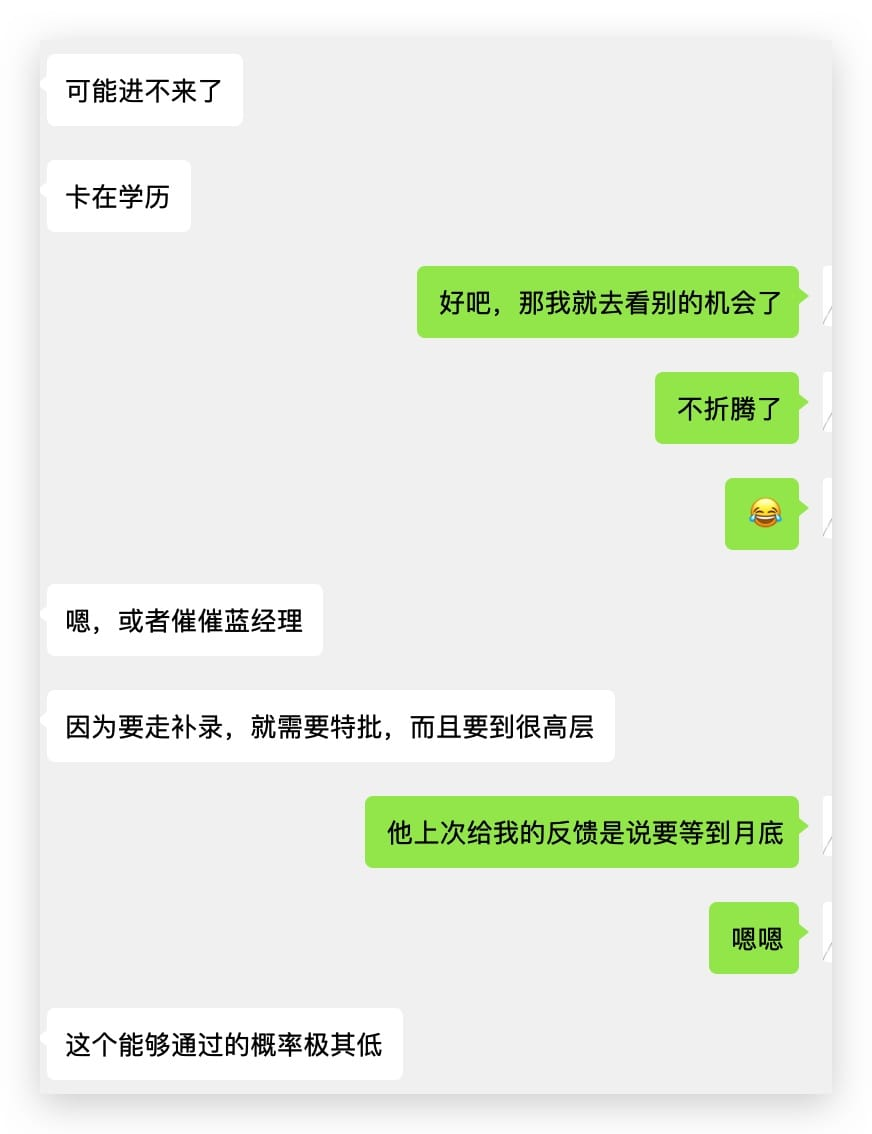## 向世界交付产品，而不是Demo

1. 一个安装文档
2. 一个配置说明
3. 一个FAQ
4. 一个升级说明（当你有 breaking changes 时，就需要写清楚升级说明，来辅助你的用户完成版本升级）

## 小程序的 marker 无法触发 bindmarkertap 事件应该如何处理

### 具体情况

``````    store.get().then(res => {
this.setData({
stores: res.data,
windowHeight: app.globalData.windowHeight,
}, () => {
wx.showToast({
title: '双指缩放可以调整地图可视区域，查看更多美食',
icon: 'none'
})
})
})``````

``````      data.map(item => {
item.id = item._id
});``````

## 如何平衡深度和广度

1. 如果你是做基础架构的，广度更重要；看得越多，知道的模式越多，基础架构越牢靠。
2. 如果你是做业务的，深度更重要；深度越深，业务越稳固。

## 低欲望年轻人

• 单人床：更大也可，但是至少应该是一张单人床
• 空调：即使是今天，我依然穿着短袖+5分裤，可以推断夏天我一定会觉得非常热，那么一个空调就有必要了。
• 能洗澡：作为一个北方来的糙汉子，公用卫生间也OK。而且我的作息和大部分互联网人不一样。12点睡 5 点起，可以避开高峰期。
• 安静：安静的房子可以让自己有更多的精力去处理自己的事情。
• 有洗衣机：虽然在大学里都是自己手洗的衣服，但是工作了以后，我可能没有太多的精力去洗衣服了。不过好消息是，按照目前的情况来看，大概率我一年四季穿 T Shirt。

## 为什么 Rails 运行 Generate 命令会很慢

TL；DR

``spring stop``

## 腾讯之行总结

TL；DR## 实现密码生成器的思路

``````function GeneratePassword() {

if (parseInt(navigator.appVersion) <= 3) {
alert("Sorry this only works in 4.0+ browsers");
return true;
}

var length=8;
length = document.aForm.charLen.options[document.aForm.charLen.selectedIndex].value;

var noPunction = (document.aForm.punc.checked);
var randomLength = (document.aForm.rLen.checked);

if (randomLength) {
length = Math.random();

length = parseInt(length * 100);
length = (length % 7) + 6
}

for (i=0; i < length; i++) {

numI = getRandomNum();
if (noPunction) { while (checkPunc(numI)) { numI = getRandomNum(); } }

}

return true;
}

function getRandomNum() {

// between 0 - 1
var rndNum = Math.random()

// rndNum from 0 - 1000
rndNum = parseInt(rndNum * 1000);

// rndNum from 33 - 127
rndNum = (rndNum % 94) + 33;

return rndNum;
}

function checkPunc(num) {

if ((num >=33) && (num <=47)) { return true; }
if ((num >=58) && (num <=64)) { return true; }
if ((num >=91) && (num <=96)) { return true; }
if ((num >=123) && (num <=126)) { return true; }

return false;
}
``````

checkPunc 则是检测输出的文字是否可以直接发音，这涉及到记忆的难度的问题，在一个长的字符串中，记忆 ABCD 肯定比记忆逗号、破折号、句号等符号要简单一些，毕竟字母肯定要好记忆点，可以自编语句来实现。

``````function GeneratePassword() {

// 检测浏览器支持
if (parseInt(navigator.appVersion) <= 3) {
alert("Sorry this only works in 4.0+ browsers");
return true;
}

var length=8;
length = 10 // 此处代码原为使用 dom 函数获取表单数据的代码，来实现自定义长度的功能。

var noPunction = false// 此处代码原为获取表单数据的代码，控制是否检测密码的可读性
var randomLength = false // 此处代码原为获取表单数据的代码，控制是否使用随机长度。

// 如果使用随机长度的密码，则进入循环
if (randomLength) {
length = Math.random();

length = parseInt(length * 100); // 生成 0 ~ 100 之间的数字
length = (length % 7) + 6 // 对生成的长度取余，则最短为6，最长为12
}

// 根据长度进行循环
for (i=0; i < length; i++) {

numI = getRandomNum();  // 获取一个随机数
if (noPunction) { while (checkPunc(numI)) { numI = getRandomNum(); } } // 如果需要判断发音，进行发音判断，判断失败就重新生成，直到成功。

}

// 设置代码到 Dom 元素中

return true;
}``````